# Part 2¶

## Getting started¶

OK, let's just dive right in and fill in details as we go. I'll be using Python for this exploration but will focus on the story and not the code.

First things first, let's load the sunspots data, which is easy to find (e.g. from NOAA) and conveniently included in a popular Python package for doing statistical work...

In :
import statsmodels.api as sm
import pandas as pd


df is shorthand for "dataframe", which we can think of as an Excel-like table of values. Dataframes have various methods that can be called to easily learn about the data contained in them, and we'll step through calling some of these methods. Below, we see that we have 309 pairs of (year, activity) to examine...

In :
df

Out:
<class 'pandas.core.frame.DataFrame'>
Int64Index: 309 entries, 0 to 308
Data columns:
YEAR           309  non-null values
SUNACTIVITY    309  non-null values
dtypes: float64(2)

We can quickly inspect the first and last handful of values to get an idea of what the data look like...

In :
df.head()

Out:
YEAR SUNACTIVITY
0 1700 5
1 1701 11
2 1702 16
3 1703 23
4 1704 36
In :
df.tail()

Out:
YEAR SUNACTIVITY
304 2004 40.4
305 2005 29.8
306 2006 15.2
307 2007 7.5
308 2008 2.9

OK, so the time series of annual values starts in 1700 and goes through 2008. Notice that we have fractional numbers in the more recent observations. This got me wondering when these fractional values started appearing...

In :
fractional_nums = df['SUNACTIVITY'].apply(lambda x: x % 1) #Take the modulo of each value with 1 to get the fractional part

Out:
49    0.9
50    0.4
51    0.7
52    0.8
53    0.7
Name: SUNACTIVITY

The first fractional value occurs in 1749. I looked into this a bit and (re)learned a few things:

• Galileo first documented sunspots in the early 1600s, using his newly invented telescope
• Reliable sunspot observations begin in about 1700
• The fractional numbers are probably associated with data coming out of Zurich, Switzerland in 1749 onward
• The methodology for tallying sunspot counts has evolved, most notably in 1848 with the introduction of the Wolf number (which is not simply an integer count)
• There seems to be a fair bit of debate about how accurate the existing data are

With some context in hand regarding the data generating process, let's get back to exploring the data. We can get a quick sense of the distribution of values...

In :
print df['SUNACTIVITY'].describe()

count    309.000000
mean      49.752104
std       40.452595
min        0.000000
25%       16.000000
50%       40.000000
75%       69.800000
max      190.200000


...and of course, any time we report statistics we should try to provide an accompanying visualization (and vice versa)...

In :
df.plot(x='YEAR', y='SUNACTIVITY', xlim=(1700,2008))

Out:
<matplotlib.axes.AxesSubplot at 0xada0550>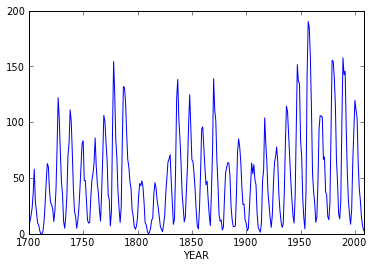Clearly there is a seasonal pattern in activity. If our aim was to fit a model to these data we would want to start looking at the autocorrelation and partial autocorrelation functions...

In :
pd.tools.plotting.autocorrelation_plot(df['SUNACTIVITY'])

Out:
<matplotlib.axes.AxesSubplot at 0xaf5e240>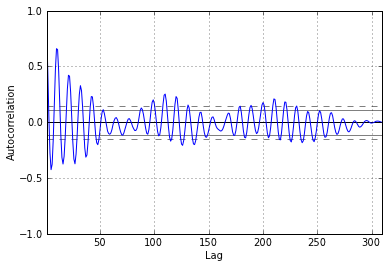That the blue line goes beyond the horizontal grey lines indicates that this is a non-stationary time series with correlation structure. For my purposes here, I am not interested in fitting a model to the data. Instead, I just want to learn more about the length of the typical sunspot cycle. For this need it helps to view the time series' autocorrelation function in the frequency domain instead of the temporal domain. So, we create an array of frequencies to evaluate the series over...

In :
import numpy as np
N2 = df.shape / 2
freqs = np.linspace(0, 0.5, num=N2, endpoint=False)[1:] #Nyquist range


...then, using these frequencies, plot the periodogram, which is the frequency domain analog of the autocorrelation plot above. Note that the Lomb-Scargle method used below assumes that the frequencies are not in a typical unit like Hertz (cycles per second) but rather as angular frequencies, which is why we need to multiply the values by $2\pi$. (The Lomb-Scargle method is flexible in that the time series need not be uniformly sampled.)

In :
import scipy as sp
periodogram = sp.signal.lombscargle(df['YEAR'], df['SUNACTIVITY'], freqs * 2 * np.pi)
plt.plot(freqs, periodogram, color='green')

Out:
[<matplotlib.lines.Line2D at 0xafd97f0>]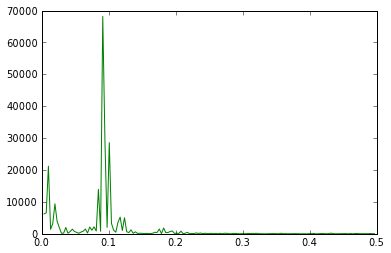We see a big spike in the power of the series at a frequency of just below 0.1. Recall that these are yearly observations, so we can divide this frequency into 1 to get the period of each cycle...

In :
freq_index_at_max_power = np.argmax(periodogram)
print 'Frequency and corresponding time in years at max power: %.2f, %.1f' % (freqs[freq_index_at_max_power], 1 / freqs[freq_index_at_max_power])

Frequency and corresponding time in years at max power: 0.09, 11.0


The major cycle is about 11 years, which is what the literature states. So, we could have skipped this previous step entirely and just assumed the data had an 11 year cycle like the literature said, but it is always good to sanity check what you are working with, and of course, in many settings one does not already know such things, hence the need for exploration.

At this point, after a handful of lines of code and some internet searches we have a basic handle on:

• The data generating process
• Distributional information
• Temporal behavior

There are a lot of things we could dive into further, but now comes the question that ties back to the title of this post: was our basic line plot of the time series data as helpful as it could be? After all, we simply plotted the data using default settings with respect to plot window size and axes scaling.

## Optimizing aspect ratio¶

In the following code segments we'll develop a method for finding an optimal aspect ratio of the plot for the sunspots data (my use of the indefinite article rather than "the optimal" is purposeful: we just want to improve upon the default plot size and not necessarily find the truly best size). The code will follow the notation that Cleveland uses in the The Details of Banking to 45$^\circ$ section in .

The first thing we do is set up the vertical and horizontal range of the data we'll be plotting...

In :
v_data = df['SUNACTIVITY'].max() - df['SUNACTIVITY'].min()
h_data = df['YEAR'].max() - df['YEAR'].min()
v_data_diffs = df['SUNACTIVITY'].diff().apply(np.abs)
vbar_data_diffs = v_data_diffs / v_data
h_data_diffs = df['YEAR'].diff().apply(np.abs)
hbar_data_diffs = h_data_diffs / h_data


Next, we'll define our objective function that we want to optimize. This function gets evaluated for each aspect ratio we want to test, and for each evaluation it calls a few supporting functions...

In :
def objective_fcn(width_height, target):
dev = setup_device_coords(figsize=width_height)
lengths = segment_lengths(dev['aspect ratio'], dev['horizontal_device'])
weighted_avg_banking =  np.sum(segment_orientations(dev['aspect ratio']) * lengths) / np.sum(lengths)
return np.abs(weighted_avg_banking - target)


The setup_device_coords function maps data coordinates to screen coordinates and calculates the vertical and horizontal range of the data in terms of their screen positions...

In :
def setup_device_coords(figsize=(8,6)):
h_device, v_device = figsize
fig, ax = plot_sunspots(figsize)
device_coords = [ax.transData.transform(data_coords) for data_coords in df.values]
df_device = pd.DataFrame(device_coords, columns=['YEAR', 'SUNACTIVITY'])
v_device = df_device['SUNACTIVITY'].max() - df_device['SUNACTIVITY'].min()
h_device = df_device['YEAR'].max() - df_device['YEAR'].min()
aspect_ratio = v_device / h_device
v_conversion = v_device / v_data
h_conversion = h_device / h_data
fig.clear()
return {'aspect ratio': aspect_ratio,
'vertical_device': v_device,
'horizontal_device': h_device,
'vertical conversion': v_conversion,
'horizontal conversion': h_conversion}


To do the data-to-screen conversion the setup_device_coords function calls a supporting function to render a plot of the data in device memory...

In :
def plot_sunspots(figsize, color='blue'):
fig = plt.figure(figsize=figsize)
fig.canvas.set_window_title('%.1f by %.1f inch window' % (figsize, figsize))
df.plot(x='YEAR', y='SUNACTIVITY', ax=ax1, linewidth=2, color=color)
fig.tight_layout()
ax1.set_xlim(right=df['YEAR'].max())
ax1.set_ylim(top=df['SUNACTIVITY'].max())
ax1.set_ylabel('Observed Sunspots')
ax1.set_title('Sunspot Activity Over Time')
return (fig, ax1)


Back to the supporting functions called by objective_fcn, we need to deteremine the lengths and slopes of each line segment in a given plot. The banking method calculates the average orientation of the line segments, where the averaging is weighted by each line segment's length.

In :
def segment_lengths(aspect_ratio, h_device):
return h_device * np.sqrt(hbar_data_diffs.dropna()**2 + aspect_ratio**2 * vbar_data_diffs.dropna()**2)

In :
def segment_orientations(aspect_ratio):
return np.arctan(aspect_ratio * vbar_data_diffs / hbar_data_diffs)


With the objective function (and its supporting functions) defined, we now need a few lines of code to drive everything. We set our desired average banking of the line segments to be 45 degrees (although the algorithms work in units of radians) and then define a grid of possible plot sizes to evaluate the objective function over. Note that the optimization method is called brute for a reason: it is a just a brute-force scan of every possible plot size, where we have defined what is possible. Since I already have experience with these data I am limiting the search to be over plotting windows that are longer than they are tall, and I am only searching over $\frac{1}{2}$ inch step-sizes in the window dimensions because we are not interested in a super-precise solution. The last line of code unpacks a list of values returned in the results variable into individual variables that we can work with directly.

In :
import scipy.optimize as spo
slice_obj = np.s_[20:26:0.5, # widths
1:4:0.5]   # heights
results = spo.brute(objective_fcn, slice_obj, args=[target], full_output=True, finish=None)
optimal_dims, objective_val, search_grid, objective_grid = results


With the optimal plot size determined, let's compare how the time series plot looks using the default aspect ratio versus the optimized one...

In :
plt.close('all')
ax1 = plot_sunspots((8,6))
print '\n\nWeighted-average banking using default 8 x 6 inch plot window: 87.3 degrees (goal is 45 degrees)'

Weighted-average banking using default 8 x 6 inch plot window: 87.3 degrees (goal is 45 degrees)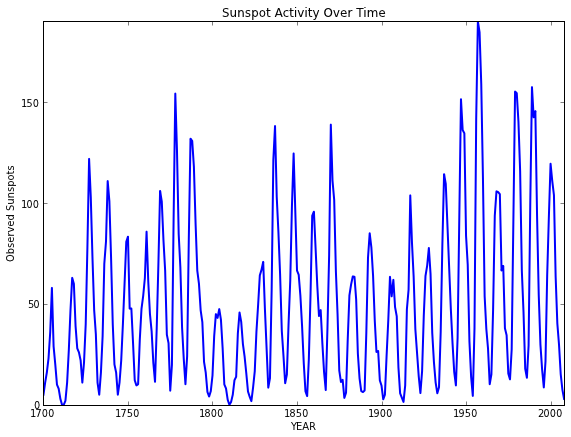This is the same plot we saw above, but bigger and with axes labels. The default aspect ratio leaves us with line segments that have an average orientation of nearly vertical, so this is a perfect example of the type of problem Cleveland was researching: It is very difficult to perceive patterns in the data when the rates of change over small chunks of time are so extreme. About all we can say is "there are cycles roughly every 10 years".

Now let's look at the same data plotted using an aspect ratio that makes the average line segment have an absolute orientation of 45 degrees...

In :
ax2 = plot_sunspots(optimal_dims, color='red')
print '\n\nOptimal width and height found to be %.1f by %.1f inches' % (optimal_dims, optimal_dims)
banking = [np.degrees(target - objective_val),
np.degrees(target + objective_val)]
print 'Average banking interval at optimized aspect ratio: (%.2f, %.2f)' % (banking, banking)

Optimal width and height found to be 25.0 by 1.5 inches
Average banking interval at optimized aspect ratio: (44.91, 45.09)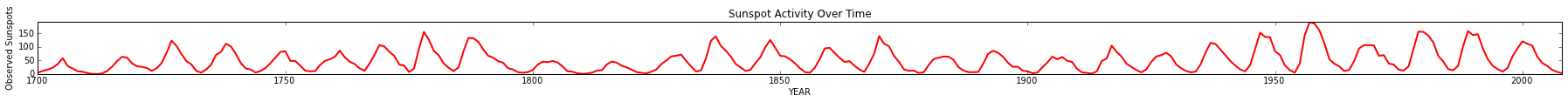(When I run this same code on my laptop I get an optimal width of 22.5 inches by the same 1.5 inch height.)

Ah, now we see something entirely new: when there are large spikes in activity the ramp up period is asymmetric with the ramp down period. Specifically, activity ramps up very quickly and tapers off more gradually. In contrast, during weaker cycles the pattern is more symmetric. This nonlinear behavior is interesting and highly studied. But, we might never investigate further had we simply plotted the data in a naive way and moved on to something else.

## Lessons¶

1. Even the most pedestrian data task, like plotting an array of values, still requires careful thought if the aim is gaining insight. Without such thought it is remarkably easy to have one's work amount to little more than generating chart porn for PowerPoint decks

2. Default settings in visualization tools are there for expediency. We will encounter plenty of opportunities that warrant going beyond these defaults to instead put intention behind our graphical results

## Extensions¶

The exploration presented here was a quick first take and there a couple of places we could improve upon. First, the method that Cleveland developed ~ 20 years ago has seen extensions, such as multiscale banking. Second, the optimization method was easy to use and understand, but a more general, faster-converging approach is certainly possible.

This entire post (executable code, results, surrounding text, and text formatting) was created using the IPython Notebook, an amazing extension of IPython that supports highly interactive, collaborative, and reproducible numerical computing. For the many cases in which I want my code to be linked with rich context, it is hard to see not using the Notebook. If your use case is focused more on text, with a bit of code interlaced, then using tools like Sweave in R or Pweave in Python are excellent options for supporting transparent, reproducible work (John Cook has a nice blog post about this nuanced difference in use cases). In either case, there are no longer excuses for not tightly coupling code, analytical results, and context :)